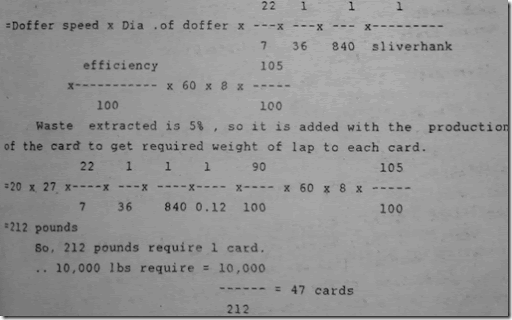### Carding Calculations in Spinning Mill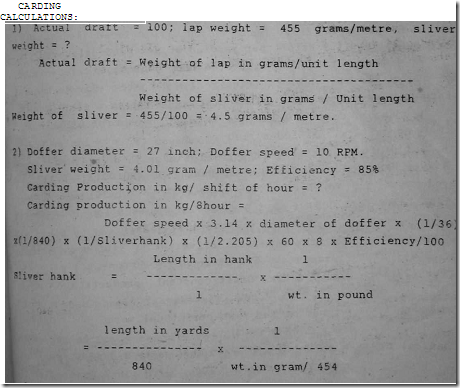Sliver wt. = 4. 01 gram / metre.

= length in yards/840 x 454/weight in gram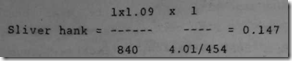production / card / Shift of 8 hours in kg.

= 10 x 3. 14 x 27 x (1/36) x (1/840) x (1/0. 147)x(1/2. 205) x 60 x 8 x (85/100) = 35. 3 kg / 8 hour

3) Lap Hank = 0. 0013; Mechanical Draft = 85; Waste = 6%; Doffer
Speed = 20 RPM; Efficiency = 85%; Production/8 hr = ?

Actual draft of card = SliverHank / Lap Hank

The removal of waste during the operation, reduces the quantity of material passing through and there is less to be delivered.

Actual draft = Weight per yardfed/wt. per yard delivered Mechanical draft (is calculated from gearing) is determined, by use of the actual draft (required) and waste%. So, Mechanical draft is always less than actual draft.

Mechanical draft = Actual draft x ((100-waste%) / 100) 85 = Actual draft x (100-6)/100 Actual draft = 85 x (100/94) = 90. 4

Sliver Hank = Actual draft x Lap Hank = 90. 4x0. 0013 = 0. 117 Production in kg/8hr. = 20 x 3. 14 x 27 x (1/36) x (1/840) x(l/0. 117) x (1/2. 205) x 60 x 8 x (85/100) = 88. 8 kg.

4) Lap weight = 400 gram / metre: Sliver wt. = 4. 05 gram / met

DCW = 17 T: waste = 6 %: efficiency = 90 % Doffer (27 inch dia) = 8 RPM. Draft constant, production ?

Actual draft = wt per mt fed / wt per mt delivered

= 400 / 4. 05 = 98. 7

Mechanical draft = actual draft x ( 100 - waste % )/ 100

= 98. 7 x (100-6) / 100 = 92. 8

Draft constant = DCW x mechanical draft 17 x 92. 8 = 1578

Sliver wt = 4. 05 gram / mt sliver hank = (length in yard / 840) x 454 / wt in gram
= 1. 09/840 x 454 / 4. 05 = 0. 145

Product ion in Kg/8 hour of carding= 8 x 3. 14 x 27 xl/36 x 1/840 x 1/0. 145 x 1/2. 205

x 60 x 8 x 90/100 = 30 Kg/8 hour.

5) Sliver Hank = 0. 15; Lap weight = 392 gram/metre

Doffer Dia = 27 inch; waste = 3% Plate wheel = 120T Side shaft bevel = 34T; Doffer wheel = 34T; Peed Roll Dia

=2. 25 inch

Draft change wheel = ?

Draft Change wheel = Draft constant/Mech. draft

Mech. draft = Actual draft x (100 - waste %)/100

Actual draft = Hank delivered/Hank fed = 0. 15/0. 0015 =100

Lap weight = 392 gram/met.

Lap Hank = (length in yard/840) x (454/wt. in gm)

= (1 x 1. 09)/840 x (454/392) = 0. 0015 Mech. draft = 100 x (100-3)/100 = 97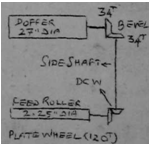To find Draft constant, let we assume The speed of the feed roller is kept as 1 Draft constant

= 1 x (120/DCW) x (34/34) x (27/2. 25)

= 1440

Draft Change Wheel = 1440/97 = 14. 3T

6) Height of Empty can = 6 kg; wt. of Pull can = 14kg Sliver Hank = 0. 14; Doffer (27 inch Dia) = 18 RPM; Efficiency = 80% Time Required to fill one can =? Sliver weight = Full can wt. - Empty can, wt. =14-6=8 kg.

To calculate the time Required to fill one can or to produce 8 kg (Production) is,

= Doffer Speed x Doffer Dia x 3. 14 x 1/36 x 1/840 x 1/Sliver Hank x 1/2. 205 x Time Requirement to produce 8 kg x Efficiency/100

Time requirement to fill one can (To produce 8 kg) = 8 x (1/Doffer speed) x (1/Doffer dia) x (1//3. 14) x

(36 x 840) x (Sliver Hank) x (2. 205) x (100/Efficiency) = 8 x 1/18 x 1/27 x 1/3. 14 x 36 x 840 x 0. 14 x 2. 205 x 100/8O = 61 Min.

7) Draft Constant = 2100; DCW = 21T; Waste = 6%

Lap fed =14 kg/40 yard; Doffer (27 inch dia) = 13 RPM Efficiency = 85%; Production= ? Lap weight = 14kg/40yard

Lap hank = (Length in yard/840) x (454/wt in gram) = (40/840) x (454/14000)=0. 0015 Mech. draft = Draft constant/DCW = 2100/21 =100 Actual draft = Mech. draft x [100/(100-waste %)]

= 100. X 100/94 =106 sliver hank = Actual draft x lap hank = 106 x. 0015 = 0. 159 i. e.. Production / 8 hour in kg =13 x 27 x (22/7) x (1/36) X (1/840) x (1/0. 159) x 60 X 8 x 85/100 x 1/2. 205 =42. 4 kg/8 hour

8)Calculate the time taken for a card to exhaust a Lap with following datas.

Lap weight = 35lbs; Sliver Hank = 0. 16; Efficiency = 85 % Waste 1 lbs: Doffer(27"0)=lO RPM Sliver weight = (lap wt- waste) =35-1= 34 pound i. e., production of the sliver from 35 lbs lap is 34 lbs. production in lbs (34) at 85% efficiency.

=Doffer speed X Dia. of doffer x 3. 14 x( 1/36) x (1/840) x (1/sliverhank) x 85/100

x time to produce 34 lbs (or) Time to exhaust one lap.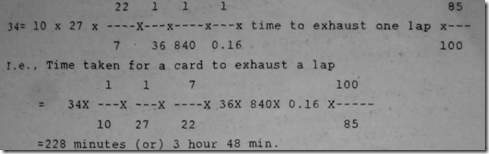9) In a blow room the production is 10. 000 lbs/shift of 8 hour calculate the number of cards you will allocate with the given details.

Doffer speed = 20 rpm Efficiency = 90%

Sliver hank = 0. 12 Doffer diameter = 27 inch

Waste = 5 % Solution:

Total lap weight requirement per card per 8 hours